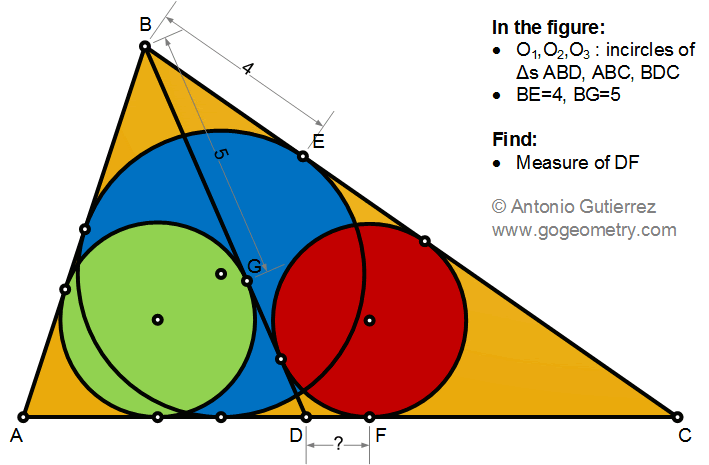## Saturday, December 5, 2020

### Geometry Problem 1490: Triangle, Cevian, Incircle, Tangent, Measurement

Geometry Problem 1490. Post your solution in the comment box below.
Level: Mathematics Education, K-12 School, Honors Geometry, College.

Details: Click on the figure below.#### 4 comments:

1.Let the semi- perimeters of Tr.s ABC, ABD & CBD be s, s1, s2 respectively. Let BD = u and AD = v and let DF = x

We have s1 + s2 = (u+v+c)/2 + (u+b-v+a)/2 = s + u ........(1)

Now s = b + 4, s1 = v + 5 and s2 = (b - v) - x + u and substituting in (1)
{v + 5} + {(b - v) - x + u} = {b + 4} + u

Hence x = 5 - 4 = 1

Sumith Peiris
Moratuwa
Sri Lanka

2.Name T, S tg points to red circle, => BT=BS
=> 5+GT=4+ES => 1+GT=ES => Name M, P tg points on AC for green, blue circles
1+GT=ES => 1+GD-TD=PD+DF => 1+1+PD-DF=PD+DF => 2=2.DF => 1=DF

1.https://photos.app.goo.gl/VGgbe9FsEAsgNYtT8

3.https://photos.app.goo.gl/os4QEybEDGrEDaW3A
Let 2p1, 2p2,2p are perimeters of triangles ABD, BDC and ABC
Note that 2p1+2p2= 2p+2.BD …..(1)
We have DF= p2-BC
HK= BK-BH= p1-BD-(p-AC)…. (2 )
Replace p1-p= BD-p2 of (1) in (2) we have
HK= -p2+BD+DC… (3)
Replace BD+DC= 2p2-BC in (3)
HK= p2-BC= DF
But HK=BK-BH=BG-BE=5-4=1